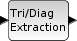Scilab Home page | Wiki | Bug tracker | Forge | Mailing list archives | ATOMS | File exchange
Change language to: English - Français - Português - 日本語 -

See the recommended documentation of this function

# EXTTRI

Triangular or Diagonal Extraction

### Block Screenshot### Description

The EXTTRI block extracts some elements from the input matrix u. The size of the output matrix is the same that those of the input matrix.

When the extraction type parameter is set to:

• 1 (Upper triangle): the block copies the elements on and above the main diagonal to an output matrix. The elements below the main diagonal are set to zero.

• 2 (Lower triangle): the block copies the elements on and below the main diagonal to an output matrix. The elements above the main diagonal are set to zero.

• 3 (Diagonal): the block copies the elements on the main diagonal to an output matrix. The elements above and below the main diagonal are set to zero.

Les fonctions équivalentes de EXTTRI dans Scilab sont respectivement triu(u), tril(u) et diag(u)

### Dialog box• Datatype(1=real double 2=Complex)

It indicates the type of the output. It support only the two types double (1) and complex (2). If we input another entry in this label xcos will print the message "Datatype is not supported".

Properties : Type 'vec' of size 1.

• Extraction type (1=lower 2=upper 3=diagonal)

It indicates the form of the output matrix. It can be an upper triangle, a lower triangle or a diagonal matrix.

Properties : Type 'vec' of size 1.

### Example

```A=[1 2 3;4 5 6;7 8 9;10 11 12]
If the extraction type is 2 then the output is
B=[1 0 0;4 5 0;7 8 9;10 11 12]```

### Default properties

• always active: no

• direct-feedthrough: yes

• zero-crossing: no

• mode: no

• regular inputs:

- port 1 : size [-1,-2] / type 1

• regular outputs:

- port 1 : size [-1,-2] / type 1

• number/sizes of activation inputs: 0

• number/sizes of activation outputs: 0

• continuous-time state: no

• discrete-time state: no

• object discrete-time state: no

• name of computational function: extrilz

### Interfacing function

• SCI/modules/scicos_blocks/macros/MatrixOp/EXTTRI.sci

### Computational function

• SCI/modules/scicos_blocks/src/c/exttril.c

• SCI/modules/scicos_blocks/src/c/exttriu.c

• SCI/modules/scicos_blocks/src/c/extdiag.c

• SCI/modules/scicos_blocks/src/c/exttrilz.c

• SCI/modules/scicos_blocks/src/c/exttriuz.c

• SCI/modules/scicos_blocks/src/c/extdiagz.c